# HBSE 10th Class Maths Solutions Chapter 13 Surface Areas and Volumes Ex 13.2

Haryana State Board HBSE 10th Class Maths Solutions Chapter 13 Surface Areas and Volumes Ex 13.2 Textbook Exercise Questions and Answers.

## Haryana Board 10th Class Maths Solutions Chapter 13 Surface Areas and Volumes Ex 13.2

Unless stated otherwise, take π = $$\frac{22}{7}$$.

Question 1.
A solid is in the shape of a cone standing on a hemisphere with both their radii being equal to 1 cm and height of the cone is euqal to its radius. Find the volume of the solid in terms of it.
Solution.
We have,
Radius of the hemisphere (r) = 1 cm
Radius of the cone (r) = 1 cm
Height of the cone (h)=1 cm
Volume of the solid = Volume of the hemisphere + Volume of the cone
= $$\frac{2}{3}$$ πr3 + $$\frac{1}{3}$$ πr2h
= $$\frac{1}{3}$$ π2 (2r + h)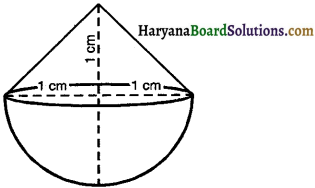= $$\frac{1}{3}$$ π × 12 (2 × 1 + 1)
= $$\frac{1}{3}$$ π × 1 × 3
Hence, volume of the solid = π cm3Question 2.
Rachel, an engineering student, was asked to make a model shaped like a cylinder with two cones attached at its two ends by using a thin aluminium sheet. The diameter of the model is 3 cm and its length is 12 cm. If each cone has a height of 2 cm, find the volume of air contained in the model that Rachel made. (Assume the outer and inner dimensions of the model to be nearly the same)
Solution:
We have,
Radius of two conical parts (r) = $$\frac{3}{2}$$ = 15 cm
Radius of the cylindrical part (r) = 15 cm
Height of the conical part (h) = 2 cm
Height of the cylindrical part (H) = 12 – (2 + 2) = 8 cm.
Volume of the air contained in the model = Volume of the cylindrical part + Volume of two conical parts
= πr2H + $$\frac{2 \times \pi r^2 h}{3}$$
= πr2 [H + $$\frac{2 \times h}{3}$$]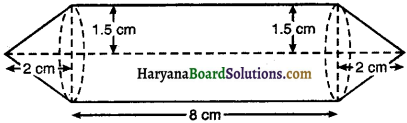= π × 1.52 [8 + $$\frac{2 \times 2}{3}$$]
= π × 2.25 × $$\frac{28}{3}$$
= $$\frac{22}{7}$$ × 2.25 × $$\frac{28}{3}$$
Hence, volume of the air contained in the model = 66 cm3Question 3.
A gulab jamun, contains sugar syrup up to about 30% of its volume. Find approximately how much syrup would be found in 45 gulab jamuns, each shaped like a cylinder with two hemispherical ends with length 5 cm and diameter 2.8 cm. (see in figure.)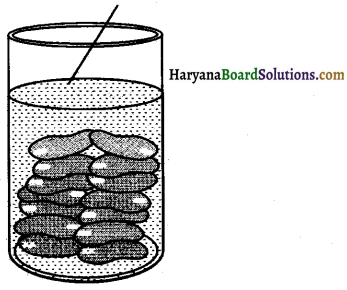Solution.
We have,
Radius of a cylindrical part (r) = 1.4 cm
Radius of hemispherical part (r) = 1.4 cm
Length of the cylindrical part of a gulab jamun (h) = [5 – (1.4 + 1.4)]
= 5 – 2.8 = 2.2 cm
Volume of gulab jamun = Volume of the cylindrical part + Volume oftwo hemispherical part
= πr2h + 2 × $$\frac{2}{3}$$πr3
= πr2 (h + $$\frac{4}{3}$$r)
= $$\frac{22}{7}$$ × (1.4)2 (2.2 + $$\frac{4}{3}$$ × 1.4)
= 6.16 × 4.0666 = 25.05 cm3
Volume of 45 gulab jamuns = 25.05 × 45 = 1127.25 cm3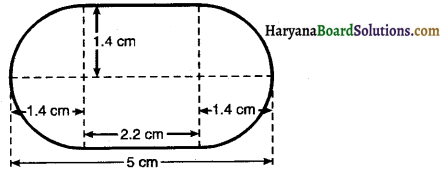Volume of the syrup = 30% of 1127.25
= $$\frac{30}{100}$$ × 1127.25 = 338.18 cm3
Hence, volume of the syrup = 338 cm3 (approx).Question 4.
A pen stand made of wood is in the shape of a cuboid with four conical depressions to hold pens. The dimensions of the cuboid are 15 cm by 10 cm by 3.5 cm. The radius of each of the depressions is 0.5 cm and the depth is 1.4 cm. Find the volume of wood in the entire stand, (see in figure.)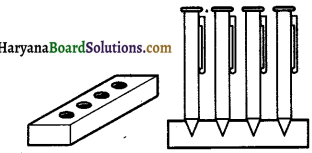Solution.
We have,
Dimensions of the cuboidal part are 15 cm × 10 cm × 3.5 cm
Volume of the cuboid = 15 × 10 × 3.5 = 525 cm3
Radius of each conical depression (r) = 0.5 cm
Depth (height) of each conical depression (h) = 1.4 cm
Volume of wood taken out to make four cavities = 4 × volume of conical depression
= 4 × $$\frac{1}{3}$$ πr2h
= 4 × $$\frac{1}{3} \times \frac{22}{7}$$ × 0.52 × 1.4
= $$\frac{88 \times 0.25 \times 0.2}{3}$$
= 1.47 cm3 (approx)
Volume of the wood in the entire stand = 525 – 1.47 = 523.53 cm3
Hence, volume of the wood in the entire stand = 523.53 cm3Question 5.
A vessel is in the form of an inverted cone. Its height is 8 cm and the radius of its top, which is open, is 5 cm. It is filled with water up to brim. When lead shots, each of which is a sphere of radius 0-5 cm are dropped into the vessel, one fourth of the water flows out. Find the member of lead shots dropped in the vessel.
Solution.
We have,
Radius of the base of the cone (r) = 5 cm
Height of the cone (h) = 8 cm
Volume of water of cone = $$\frac{1}{3}$$ πr2h
= $$\frac{1}{3}$$ × π × 52 × 8
⇒ $$\frac{200 \pi}{3}$$ cm3
Volume of the water that flows out of the cone = $$\frac{1}{4} \times \frac{200 \pi}{3}$$
= $$\frac{50 \pi}{3}$$ cm3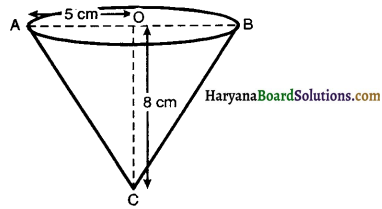Volume of spherical lead = $$\frac{4}{3} \pi r_1^3=\frac{4}{3} \pi \times(0.5)^3$$
= $$\frac{\pi \times 0.5}{3}=\frac{\pi}{6}$$ cm3
Let n spherical lead shots are dropped into the vessel.
So that $$\frac{1}{4}$$ th of the water contianed in the vessel flows out.
∴ Volume of n spherical lead shots = Volume of water that flows out
⇒ n $$\frac{\pi}{6}=\frac{50}{3} \pi$$
⇒ n = $$\frac{50 \pi}{3} \times \frac{6}{\pi}$$
⇒ n = 100
Hence, number of lead shots dropped in the vessel = 100.Question 6.
A solid iron pole consists of a cylinder of height 220 cm and base diameter 24 cm, which is surmounted by another cylinder of height 60 cm and radius 8 cm. Find the mass of the pole, given that 1 cm3 of iron has approximately 8 g mass. (Use π = 3.14)
Solution.
We have,
First cylindrical part has radius 12 cm and height 220 cm
Its volume = π × 122 × 220 = 31680π cm3
Ilnd cylindrical part has radius 8 cm and height 60 cm
Its volume = π × 82 × 60 = 3840π cm3
Total volume of iron pole = 31680π + 3840π = 35520π
= 35520 × 3.14 = 111532.8 cm3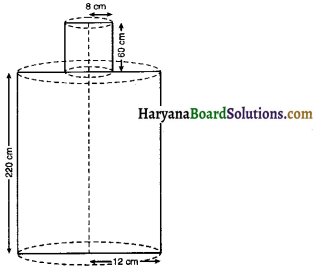Total mass of the iron pole = 111532.8 × 8 gm
= $$\frac{111532 \cdot 8 \times 8}{1000}$$ kg
= 892.2624 kg = 892.26 kg
Hence, weight of iron pole = 892.26 kg.Question 7.
A solid consisting of a right circular cone of height 120 cm and radius 60 cm standing on a hemisphere of radius 60 cm is placed upright in a right circular cylinder full of water such that it touches the bottom. Find the volume of water left in the cylinder, if the radius of the cylinder is 60 cm and its height is 180 cm.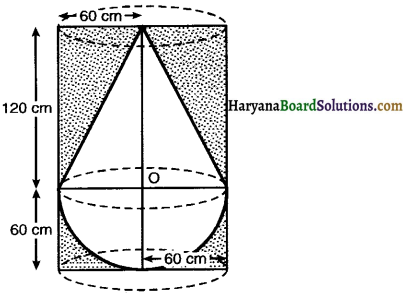Solution:
We have,
Radius of the cylinder (r) = 60 cm
Height of the cylinder (H) = 180 cm
Volume of water that the cylinder contain = πr2h
= π × 602 × 180
= 648000π cm3
Radius of the conical part (r) = 60 cm
Radius of the hemispherical part (r) = 60 cm
Height of the conical part (h) = 120 cm
Volume of the solid = Volume of conical part + Volume of hemispherical part
= $$\frac{1}{3}$$ πr2h + $$\frac{2}{3}$$ πr3
= $$\frac{1}{3}$$ πr2 (h + 2r)
= $$\frac{1}{3}$$ π × 602 (120 + 2 × 60)
= $$\frac{1}{3}$$ π × 3600 × 240
= 288000π cm3
Volume of water left in the cylinder = Volume of water that cylinder contains – Volume of solid
= 648000 π – 288000 π
= 360000π
= 360000 × $$\frac{22}{7}$$ cm3
= $$\frac{360000 \times 22}{7 \times 100 \times 100 \times 100}$$ m3
[∵ 1 cm3 = $$\frac{1}{100} \times \frac{1}{100} \times \frac{1}{100}$$ m3]
= $$\frac{22 \times 36}{700}$$ m3
= 1.1314 m3
= 1.131 m3 (approx)
Hence, Volume of water out in the cylinder = 1.131 m3 (approx).Question 8.
A spherical glass vessel has a cylindrical neck 8 cm long, 2 cm in diameter ; the diameter of the spherical part is 8.5 cm. By measuring the amount of water it holds, a child finds its volume to be 345 cm3. Check whether she is correct, taking the above as the inside measurements and π = 3.14.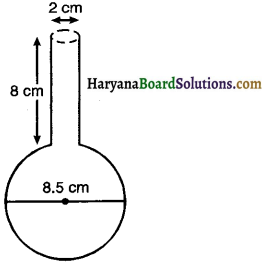Solution.
We have,
Radius of cylindrical neck (r) = $$\frac{2}{2}$$ = 1 cm
Height of cylindrical neck (A) = 8 cm
Radius of the spherical part (R) = $$\frac{8.5}{2}$$ cm
Amount of water in the vessel = Volume of the cylindrical neck + Volume of the spherical part
= πr2h + $$\frac{4}{3}$$ πR3
= π(r2h + $$\frac{4}{3}$$ R3)
= 3.14 [12 × 8 + $$\frac{4}{3} \times\left(\frac{8 \cdot 5}{2}\right)^3$$]
= 3.14 (8 + 102.354)
= 3.14 × 110.354
= 346.51 cm3
Hence, volume find by the child is not correct.